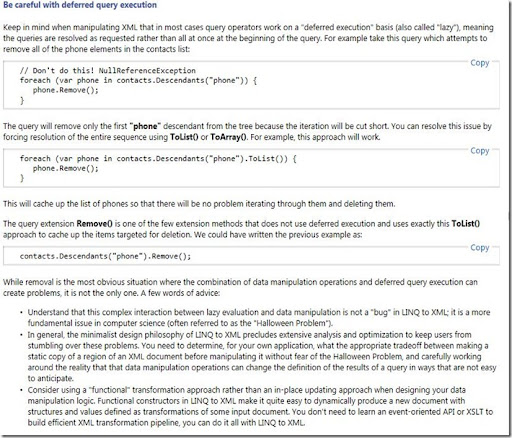## Wednesday, June 22, 2011

### LINQ to XML, when lazy is too lazy in F# (and C# )

Today a F# program did not work as expected. This is a small example I created:

```let xml ="<a><b>1</b><b>2</b></a>"
let doc = XDocument.Parse(xml)

let getXElements_b (doc:XDocument) =
let els = doc.Root.Elements(XName.Get("b"))
els```
```//test
let result1 = doc |> getXElements_b |> Seq.toList
//val result1 : XElement list = [<b>1</b>; <b>2</b>]
//the expected result ```
```
let proces (el:XElement) =
XElement(XName.Get("c")) |> el.ReplaceWith

doc |> getXElements_b |> Seq.iter proces

let result2 =  doc.ToString();;
//val result2 : string = "<a>
//  <c />
//  <b>2</b>
//</a>"
```
This is not the expected result. I expected:

```val result2 : string = "<a>
<c />
<c />
</a>"
```

I can fix it by changing the getXElements_b function:

```let getXElements_b (doc:XDocument) =
let els = doc.Root.Elements(XName.Get("b")) |> Seq.toList
els
```

With this adjustment everything works fine. It seems that I have to force the the computation.

This is a problem in C# too.

```private void GetXml()
{
var xml = "<a><b>1</b><b>2</b></a>";
var doc = XDocument.Parse(xml);
var els = doc.Root.Elements(XName.Get("b"));

foreach (var el in els)
{
el.ReplaceWith(new XElement(XName.Get("c")));
}

txtResult.Text = doc.ToString();
}```
`produces:`

<a>
<c />
<b>2</b>
</a>

When I step with the debuger through the code the foreach is only one time triggered.

With support of Google I found the following document:

http://msdn.microsoft.com/en-us/library/bb308960.aspxYou can learn something new everyday.